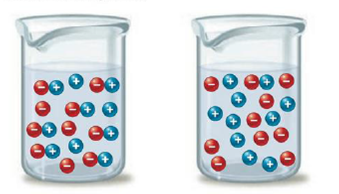Chapter 11, Problem 84E

Chapter
Section
Textbook Problem

# Consider the following representations of an ionic solute in water. Which flask contains MgSO4, and which flask contains NaCl? How can you tell?Interpretation Introduction

Interpretation: To predict the flask contains MgSO4 and NaCl .

Concept introduction:

The relationship among the moles of solute dissolved as well as the moles of particles

in solution is frequently articulated using the van't Hoff factor.

i = molesofparticlesinsolutionmolesofsolutedissolved

Explanation

To find the figure on the left side represents the MgSO4 solution.

MgSO4 has an experimental i value of 1.3 whereas the observed i value for NaCl is 1.9 . Both salts have a usual i value of 2. The usual i value for a salt is determined by assuming 100% of the salt breaks up keen on divide cations and anions

### Still sussing out bartleby?

Check out a sample textbook solution.

See a sample solution

#### The Solution to Your Study Problems

Bartleby provides explanations to thousands of textbook problems written by our experts, many with advanced degrees!

Get Started